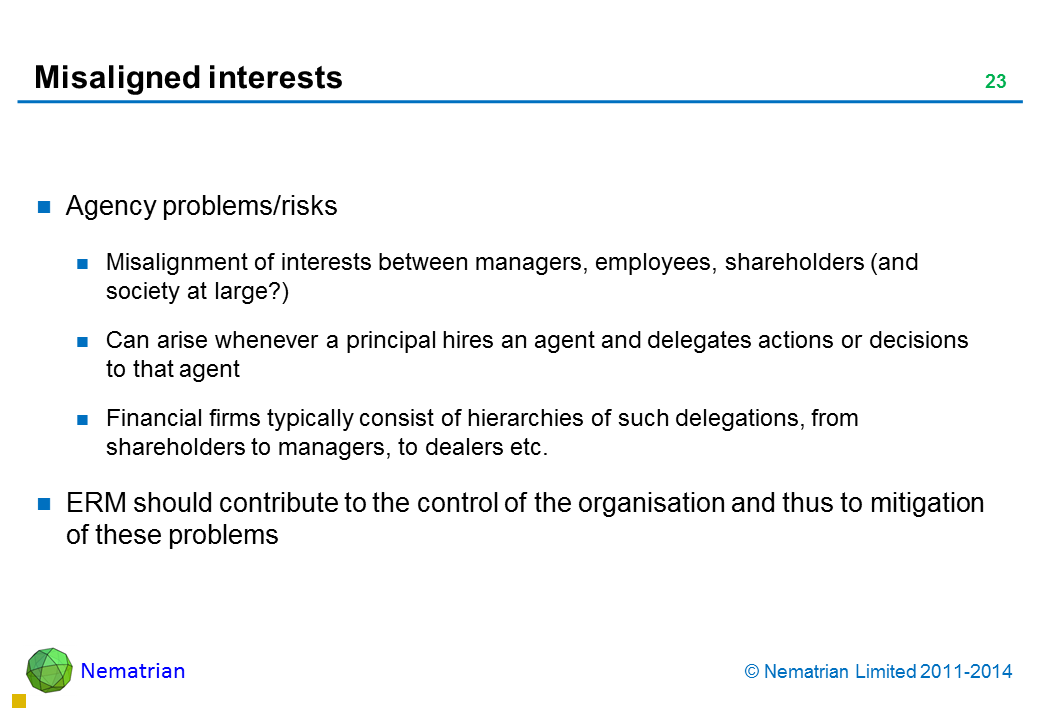/

### var x2=document.getElementById("RandomWalkHyperlink"); x2.setAttribute("width","250"); x2.setAttribute("height","30"); x2.setAttribute("style","border: 1px solid black"); var c2=x2.getContext("2d"); var sigma=.06; var mu=0; var y=new Array; var r=new Array; var ylength=100; var i; var xplotlow=2; var xplothigh=80; var yplotlow=x2.height-2; var yplothigh=6; var frameRate=100; var lineColour="red" y=1; for (i=1;i<ylength;i++) { r[i]=inverseNormal(Math.random()); y[i]=y[i-1]*Math.exp(mu+sigma*r[i]); } projectLineChartOntoCanvas(c2,y,xplotlow,xplothigh,yplotlow,yplothigh,lineColour); animateRandomWalk(x2,c2,y,xplotlow,xplothigh,yplotlow,yplothigh,lineColour,mu,sigma,frameRate) function animateRandomWalk(canvas,ctx,y,xplotlow,xplothigh,yplotlow,yplothigh,strokeStyle,mu,sigma,frameRate) { var i; var plotID=null; plotIncrement(); plotID=setInterval(plotIncrement,frameRate); function plotIncrement() { ctx.fillStyle="white"; ctx.fillRect(0,0,canvas.width,canvas.height); ctx.clearRect(0,0,canvas.width,canvas.height); var i; var ylength=y.length; for (i=0;i<ylength-1;i++) { y[i]=y[i+1]; } y[ylength-1]=y[ylength-2]*Math.exp(mu+sigma*inverseNormal(Math.random())); ctx.beginPath(); projectLineChartOntoCanvas(ctx,y,xplotlow,xplothigh,yplotlow,yplothigh,strokeStyle); c2.fillStyle="maroon"; c2.font="16px Arial"; c2.fillText("ERM Resources",110,21); } } function projectLineChartOntoCanvas(ctx,y,xplotlow,xplothigh,yplotlow,yplothigh,strokeStyle) { var i,ylow,yhigh,xplotscale,yplotscale; var xplot=new Array; var yplot=new Array; ylength=y.length; ylow=0; yhigh=0; for (i=0;i<ylength;i++) { if (y[i]<ylow) { ylow=y[i];} if (y[i] > yhigh) { yhigh=y[i];} } xplotscale=(xplothigh-xplotlow)/(ylength-1); yplotscale=(yplothigh-yplotlow)/(yhigh-ylow); for (i=0;i<ylength;i++) { xplot[i]=xplotlow+i*xplotscale; yplot[i]=yplotlow+y[i]*yplotscale; } ctx.beginPath(); ctx.strokeStyle=strokeStyle; ctx.moveTo(xplot,yplot); for (i=1;i<ylength;i++) { ctx.lineTo(xplot[i],yplot[i]); } ctx.stroke(); ctx.beginPath(); ctx.strokeStyle="green"; ctx.lineWidth=1; ctx.strokeRect(xplotlow,Math.min(yplotlow,yplothigh)-4,xplothigh-xplotlow,Math.abs(yplothigh-yplotlow)+4); ctx.stroke(); } function inverseNormal(x){ // Adapted from Peter Acklam's "An algorithm for computing the inverse normal cumulative distribution function" var a1=-39.6968302866538; var a2=220.946098424521; var a3=-275.928510446969; var a4=138.357751867269; var a5=-30.6647980661472; var a6=2.50662827745924; var b1=-54.4760987982241; var b2=161.585836858041; var b3=-155.698979859887; var b4=66.8013118877197; var b5=-13.2806815528857; var c1=-0.00778489400243029; var c2=-0.322396458041136; var c3=-2.40075827716184; var c4=-2.54973253934373; var c5=4.37466414146497; var c6=2.93816398269878; var d1=0.00778469570904146; var d2=0.32246712907004; var d3=2.445134137143; var d4=3.75440866190742; var x_low=0.02425; var x_high=1-x_low; var q,r,ans; if (x <= 0) { throw "InverseNormal: argument outside valid range" } else if (x<x_low) { q=Math.sqrt(-2*Math.log(x)) return (((((c1*q+c2)*q+c3)*q+c4)*q+c5)*q+c6)/ ((((d1*q+d2)*q+d3)*q+d4)*q+1) } else if (x <= x_high) { q=x-0.5 r=q*q return (((((a1*r+a2)*r+a3)*r+a4)*r+a5)*r+a6)*q/ (((((b1*r+b2)*r+b3)*r+b4)*r+b5)*r+1) } else if (x<1) { q=Math.sqrt(-2*Math.log(1-x)) return -(((((c1*q+c2)*q+c3)*q+c4)*q+c5)*q+c6)/ ((((d1*q+d2)*q+d3)*q+d4)*q+1) } else { throw "InverseNormal: argument outside valid range" } } ERM concepts and Risk categorisation 

Go to: Summary | Previous | NextNAVIGATION LINKS
Contents | Prev | Next | ERM Lecture Series

 Software Research Consulting Calculator Publications Examples Functions ERM Education Spreadsheets References Contact us HTML/CSS/JS Other

© 2019 - Nematrian Limited

This site uses cookies to improve and monitor its performance. Cookies may already be placed on your computer. By continuing to use this site, you are agreeing to accept these cookies which can be deleted at any time.
License Agreement etc. | Disclaimer
Desktop view | Switch to Mobile